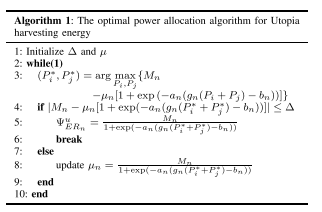# Successive convex approximation is used in my algorithm

My objective function is exponantial so i am using mosek solver. This type of error is occuring in code (“Pi” was previously used as a variable, conflicting with its use here as the name of a function or command.See “How MATLAB Recognizes Command Syntax” in the MATLAB documentation for details.)
This is my code,
while 1
count(1,i) = count(1,(i-1))+1;
cvx_begin quiet
cvx_solver mosek
variables Pi(n,1) Pj(n,1) %optimization variables
maximize(Mn-mu_n.(1+exp(-an(gnx.(Pi(1,i)+Pj(1,i))-bn))))
subject to
Pi <= Pi_opt;
Pj <= Pj_opt;
qmx.
(Pi+Pj)<= Gamma_m;
cvx_end
Pi Pj
K = abs(Mn-mu_n.(1+exp(-an.(gnx.(Pi(1,i)+Pj(1,i))-bn))));
fprintf(‘value of K:%f\n’,K);
fprintf(‘value of Pix:%f\n’,Pi(1,i));
fprintf(‘value of Pjx:%f\n’,Pj(1,i));
if K < Delta
Phi_ERn(1,i) = Mn./(1+exp(-an.
(gnx.(Pi(1,i)+Pj(1,i))-bn)));
fprintf(‘value of Phi_ERn:%f\n’,Phi_ERn(1,i));
break
else
mu_n1 = Mn./(1+exp(-an.
(gnx.*(Pi(1,i)+Pj(1,i))-bn)));
mu_n1x = abs(mu_n1);
mu_n = mu_n1x;
fprintf(‘value of updated mu_n:%f\n’,mu_n)
end
i = i+1;
end
endthis is my algorithm.

If you are getting this message, then change the function or variable name so that they don;t conflict. Do you have some other question?

Given your use of `exp`, I suggest you use CVXQUAD in order to improve reliability and performance. Please read CVXQUAD: How to use CVXQUAD's Pade Approximant instead of CVX's unreliable Successive Approximation for GP mode, log, exp, entr, rel_entr, kl_div, log_det, det_rootn, exponential cone. CVXQUAD's Quantum (Matrix) Entropy & Matrix Log related functions

Thank you so much sir, this is very helpful for me. This is my program
%convex optimization for optimizing allocated powers (P1 and P2)
cvx_begin quiet
cvx_solver mosek
variable P(2,1) %optimization variables
maximize(Mn-mu_n*(1+exp(-an*(gnx*(P(1,1)+P(2,1))-bn))))
subject to
log2(1 + (P(1).*hix)./(P(2).*hix + Ni)) >= R_min;
log2(1 + (P(2)*hjx)./Nj) >= R_min;
log2(1+(P(1)hjx)./(P(2)hjx + Nj)) >= R1+R2;
qmx
(P(1)+P(2)) <= Gamma_m;
cvx_end
P
I am getting error like wise
Error using .
(line 173)
Disciplined convex programming error:
Cannot perform the operation: {real affine} ./ {real affine}

Error in ./ (line 19)
z = times( x, y, ‘./’ );

Error in * (line 36)
z = feval( oper, x, y );

Error in / (line 15)
z = mtimes( x, y, ‘rdivide’ );

Error in nov22 (line 41)
R1 = log2(1+(P(1)*hix)/(P(2)*hix)+Ni);

Help me sir to solve it out,

if i am doing this,
%convex optimization for optimizing allocated powers (P1 and P2)

``````cvx_begin quiet
variable P(2,1)   %optimization variables
R_min = 1;   % 1 bits/Hz (minimum transmission rate)
maximize(Mn-mu_n*(1+exp(-an*(gnx*(P(1)+P(2))-bn))))
subject to
R1 = log2(1+mtimes(feval(times,P(1,1),hix),(feval(times,P(2,1),hix)+Ni),.....
'rdivide'));
R2 = log2(1+mtimes(feval(times,P(2,1),hjx),Nj,'rdivide'));
R3 = log2(1+mtimes(feval(times,P(1,1),hjx),((feval(times,P(2,1),hjx)+Nj)),.........
'rdivide'))
log2(1+mtimes(feval(times,P(1,1),hix),(feval(times,P(2,1),hix)+Ni),..........
'rdivide')) >= R_min;
log2(1+mtimes(feval(times,P(2,1),hjx),Nj,'rdivide')) >= R_min;
log2(1+mtimes(feval(times,P(1,1),hjx),((feval(times,P(2,1),hjx)+Nj)),.........
'rdivide')) >= R1+R2;
feval(times,qmx,P(1,1)+P(2,1)) <= Gamma_m;
mu_n >= 0;
cvx_end
P
``````

Getting this error
Error using .*
Not enough input arguments.

Error in abhi (line 40)
`R1 = log2(1+mtimes(feval(times,P(1,1),hix),(feval(times,P(2,1),hix)+Ni),.....`